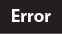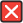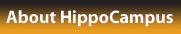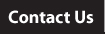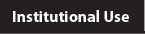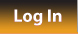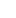More OER Help Center Log In About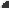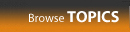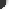Select All ContentPresentations
Phoenix College Collection
Arithmetic
Geometry
Algebra 1
Algebra 2
Trigonometry
Calculus I
Calculus II
Calculus III
Linear Algebra
Differential Equations
Financial MathematicsWorked Examples
Phoenix College Collection
Arithmetic
Geometry
Algebra 1
Algebra 2
Trigonometry
Calculus I
Calculus II
Calculus III
Linear Algebra
Differential Equations
Nursing Math
Financial MathematicsTest Prep
Phoenix College Collection
SAT Math Practice
 Search:Select an item from the left column to display a list of Topics. (To select multiple items, hold down the command or control key.)
Sequential
Topic Name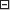hide column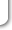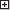maximize